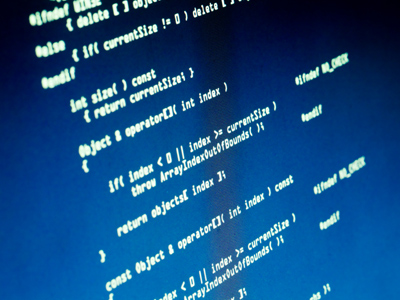See if you can crack these codes!

# Number Series 1

In this 11-plus verbal reasoning series of number quizzes you have to find the number that continues the sequence in the most sensible way.

You will be offered a sequence of numbers, and you have to find the missing number each time. Some questions will be straightforward, and you will just have to work out the relationship between the first two numbers, then between the second and third and so on. Some will be more difficult, requiring you to add different numbers in the sequence.

An example has been done for you so please make sure you read it carefully first before starting the quizzes. If you get stuck, you can always revisit the example! Good luck.

Example: What is the next number in the series of numbers below? Replace the question mark with a number and select the correct answer from the four choices available.

2, 4, 6, 8, 10 (?)

12
11
14
2

The correct answer is 12 because the numbers are going up by 2 each time. 10 + 2 = 12.
1.
What is the next number in the series of numbers below? Replace the question mark with a number and select the correct answer from the four choices available.
1, 5, 9, 13, (?)
17
19
31
71
The correct answer is 17 because the numbers are going up by 4 each time. 13 + 4 = 17
2.
What is the next number in the series of numbers below? Replace the question mark with a number and select the correct answer from the four choices available.
121, 100, 81, 64, (?)
44
49
34
48
The correct answer is 49 because the numbers are all the squares of other numbers. 11 x 11 = 121, 10 x 10 = 100 and so on. So we are looking for the square of 7 which is 49
3.
What is the next number in the series of numbers below? Replace the question mark with a number and select the correct answer from the four choices available.
2, 3, 5, 7, 11, (?)
15
14
13
19
The correct answer is 13 because the numbers are all the prime numbers in order. We are looking for the next number which can be divided only by itself and 1. Only 13 works
4.
What is the next number in the series of numbers below? Replace the question mark with a number and select the correct answer from the four choices available.
4, 16, 5, 25, 6, (?)
34
35
32
36
The correct answer is 36 because the number pattern is a number followed by its square. The next square is 6 x 6 which is 36
5.
What is the next number in the series of numbers below? Replace the question mark with a number and select the correct answer from the four choices available.
543, 432, 321, 210, (?)
92
96
99
101
The correct answer is 99 because the numbers are going down by 111 each time. 210 - 111 = 99
6.
What is the next number in the series of numbers below? Replace the question mark with a number and select the correct answer from the four choices available.
31, 37, 41, 47, (?)
48
45
50
51
The correct answer is 51 because the numbers are going up first by 6, then by 4. We are looking for 47 + 4 = 51
7.
What is the next number in the series of numbers below? Replace the question mark with a number and select the correct answer from the four choices available.
8, 12, 16, 20, (?)
22
24
26
28
The correct answer is 24 because the numbers are going up by 4 each time. 20 + 4 = 24
8.
What is the next number in the series of numbers below? Replace the question mark with a number and select the correct answer from the four choices available.
0, 1, 1, 2, 3, (?)
5
6
7
8
The correct answer is 5 because the numbers are a Fibonacci sequence. This means that each number is the sum of the two numbers to its left. So, 2 + 3 = 5. Fibonacci was an Italian mathematician whose full name was Leonardo Pisano Bigollo. He did not invent Fibonacci numbers, but used them in his Maths
9.
What is the next number in the series of numbers below? Replace the question mark with a number and select the correct answer from the four choices available.
987, 876, 765, 654, (?)
513
543
542
563
The correct answer is 543 because the numbers are going down by 111 each time. 654 - 111 = 543
10.
What is the next number in the series of numbers below? Replace the question mark with a number and select the correct answer from the four choices available.
2048, 1024, 512, 256, (?)
126
128
124
122
The correct answer is 128 because the numbers are half of the previous number each time. We use these numbers when counting the size of computer memory. You can have a megabyte (1024 bytes) or a gigabyte (1024 megabytes.) Well done! You have completed the first of our four Number Series quizzes
Author:  Stephen O'Hara